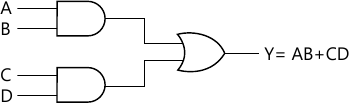MORE IN Design of Programming with Logic
VTU Computer Science (Semester 3)
Design of Programming with Logic
June 2015
Total marks: --
Total time: --
INSTRUCTIONS
(1) Assume appropriate data and state your reasons
(2) Marks are given to the right of every question
(3) Draw neat diagrams wherever necessary

1 (a) Define: i) Rise time
ii) Fall time
iii) Period and
iv) Frequency.
8 M
1 (b) What is an universal gate? List the universal gates and prove their universalities.
6 M
1 (c) Write the Verilog code for the circuit given below.6 M

2 (a) Using K-map find the reduced SOP form of
f(A, B, C, D) = ∑M(5, 6, 12, 13) + ∑d (4, 9, 14, 15)
5 M
2 (b) What is a hazard? List the types of hazards and explain static-0 and static-1 hazards.
5 M
2 (c) Simplify the following using Mc-Cluskey method.
F=∑M (0,1, 2, 8, 10, 11, 14, 15).
10 M

3 (a) Implement the following function using a 8:1 multiplexer:
f(a, b, c, d) = ∑M(0, 1, 5, 6, 8, 10, 12, 15).
8 M
3 (b) Realize the following function using the 3:8 decoder
F1(A, B, C) = ∑M (1, 2, 3, 4), F2(A, B, C) = ∑M(3, 5, 7).
6 M
3 (c) What is a magnitude comparator? Explain with a neat block diagram an n-bit magnitude comparator.
6 M

4 (a) With a neat block diagram, explain the working of a Master-Slave JK flip flop. Also write its truth table.
10 M
4 (b) Define: i) Flip flop ii) Hold time iii) Set up time iv) Characteristics equation.
4 M
4 (c) Calculate the block cycle time for a system that uses a clock, that has a frequency of
i) 10 Mhz
ii) 50 Mhz
iii) 750 Khz
6 M

5 (a) Draw the logic diagram of a 4-bit serial out shift register using J-K flip flop and explain.
8 M
5 (b) Explain briefly serial adder with a neat sketch.
8 M
5 (c) Write a Verilog code for switched tail counter.
4 M

6 (a) Briefly explain 3-bit binary ripple up-counter. Also write the truth table and waveform.
10 M
6 (b) Design a Modulo-5 up counter (synchronous) using j-k flip flop.
10 M

7 (a) With neat block diagrams compare Mealy Model and Moore model of sequential logic system.
8 M
7 (b) Draw the ASM chart for vending machine problem using Mealy model.
12 M

8 (a) Explain the concept of 'Successive approximation' of a A/D converter.
10 M
8 (b) Draw a binary ladder network for a digital input 1000 and obtain its equivalent circuit.
10 M

More question papers from Design of Programming with Logic